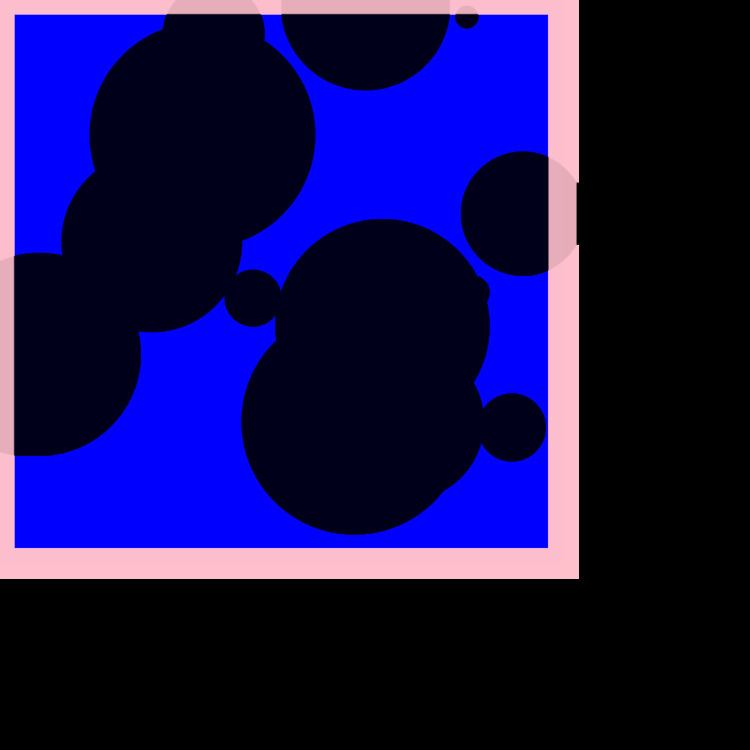Boolean model (probability theory)

Updated on
Covid-19In probability theory, the Boolean-Poisson model or simply Boolean model for a random subset of the plane (or higher dimensions, analogously) is one of the simplest and most tractable models in stochastic geometry. Take a Poisson point process of rate λ in the plane and make each point be the center of a random set; the resulting union of overlapping sets is a realization of the Boolean model B . More precisely, the parameters are λ and a probability distribution on compact sets; for each point ξ of the Poisson point process we pick a set C ξ from the distribution, and then define B as the union ξ ( ξ + C ξ ) of translated sets.

To illustrate tractability with one simple formula, the mean density of B equals 1 exp ( λ A ) where Γ denotes the area of C ξ and A = E ( Γ ) . The classical theory of stochastic geometry develops many further formulae.

As related topics, the case of constant-sized discs is the basic model of continuum percolation and the low-density Boolean models serve as a first-order approximations in the study of extremes in many models.

Boolean model (probability theory) Wikipedia

Topics